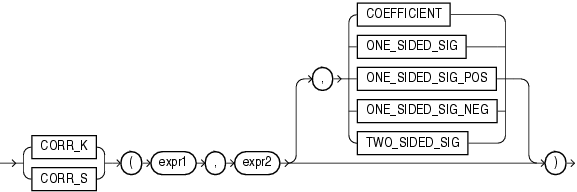## CORR_*

The `CORR_`* functions are:

• `CORR_S`

• `CORR_K`

Syntax

correlation::=Description of the illustration correlation.eps

Purpose

The `CORR` function (see CORR) calculates the Pearson's correlation coefficient and requires numeric expressions as input. The `CORR_`* functions support nonparametric or rank correlation. They let you find correlations between expressions that are ordinal scaled (where ranking of the values is possible). Correlation coefficients take on a value ranging from -1 to 1, where 1 indicates a perfect relationship, -1 a perfect inverse relationship (when one variable increases as the other decreases), and a value close to 0 means no relationship.

These functions takes as arguments any numeric data type or any nonnumeric data type that can be implicitly converted to a numeric data type. Oracle Database determines the argument with the highest numeric precedence, implicitly converts the remaining arguments to that data type, makes the calculation, and returns `NUMBER`.

• Table 2-8 for more information on implicit conversion and Numeric Precedence for information on numeric precedence

• Appendix C in Oracle Database Globalization Support Guide for the collation determination rules, which define the collation `CORR_K` and `CORR_S` use to compare characters from `expr1` with characters from `expr2`

`expr1` and `expr2` are the two variables being analyzed. The third argument is a return value of type `VARCHAR2`. If you omit the third argument, then the default is `COEFFICIENT`. The meaning of the return values is shown in the table that follows:

Table 7-2 CORR_* Return Values

Return Value Meaning

`COEFFICIENT`

Coefficient of correlation

`ONE_SIDED_SIG`

Positive one-tailed significance of the correlation

`ONE_SIDED_SIG_POS`

Same as `ONE_SIDED_SIG`

`ONE_SIDED_SIG_NEG`

Negative one-tailed significance of the correlation

`TWO_SIDED_SIG`

Two-tailed significance of the correlation

### CORR_S

`CORR_S` calculates the Spearman's rho correlation coefficient. The input expressions should be a set of (xi, yi) pairs of observations. The function first replaces each value with a rank. Each value of xi is replaced with its rank among all the other xis in the sample, and each value of yi is replaced with its rank among all the other yis. Thus, each xi and yi take on a value from 1 to `n`, where `n` is the total number of pairs of values. Ties are assigned the average of the ranks they would have had if their values had been slightly different. Then the function calculates the linear correlation coefficient of the ranks.

CORR_S Example

Using Spearman's rho correlation coefficient, the following example derives a coefficient of correlation for each of two different comparisons -- `salary` and `commission_pct`, and `salary` and `employee_id`:

```SELECT COUNT(*) count,
CORR_S(salary, commission_pct) commission,
CORR_S(salary, employee_id) empid
FROM employees;

COUNT COMMISSION      EMPID
---------- ---------- ----------
107 .735837022 -.04473016```

### CORR_K

`CORR_K` calculates the Kendall's tau-b correlation coefficient. As for `CORR_S`, the input expressions are a set of (xi, yi) pairs of observations. To calculate the coefficient, the function counts the number of concordant and discordant pairs. A pair of observations is concordant if the observation with the larger x also has a larger value of y. A pair of observations is discordant if the observation with the larger x has a smaller y.

The significance of tau-b is the probability that the correlation indicated by tau-b was due to chance—a value of 0 to 1. A small value indicates a significant correlation for positive values of tau-b (or anticorrelation for negative values of tau-b).

CORR_K Example

Using Kendall's tau-b correlation coefficient, the following example determines whether a correlation exists between an employee's salary and commission percent:

```SELECT CORR_K(salary, commission_pct, 'COEFFICIENT') coefficient,
CORR_K(salary, commission_pct, 'TWO_SIDED_SIG') two_sided_p_value
FROM employees;

COEFFICIENT TWO_SIDED_P_VALUE
----------- -----------------
.603079768        3.4702E-07```